# AP Physics 1 Multiple-Choice Practice Test 22

### Test Information10 questions18 minutes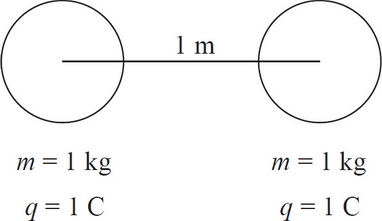1. Two identical spheres of mass 1 kg are placed 1 m apart from each other. Each sphere pulls on the other with a gravitational force, Fg. If each sphere also holds 1 C of positive charge, then the magnitude of the resulting repulsive electric force is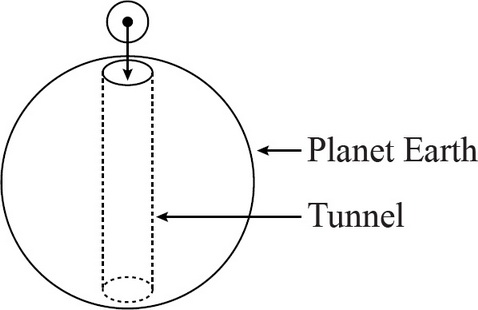2. If a hole were dug through the center of a planet and a ball dropped into the hole, which of the following best describes the motion that the ball would undergo? Assume the ball is indestructible and the planet is a perfect sphere.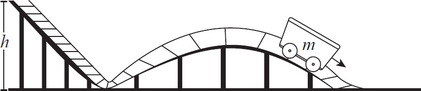3. An empty mine car of mass m starts at rest at the top of a hill of height h above the ground, then rolls down the hill and into a semicircular banked turn. Ignoring rolling friction so that the only forces acting on the mine car are the normal force from the track and gravity, what is the magnitude of centripetal force on the car as it rounds the banked curve?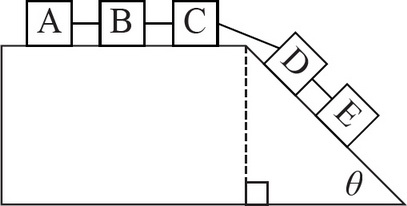4. Five boxes are linked together, as shown above. If both the flat and slanted portions of the surface are frictionless, what will be the acceleration of the box marked B ?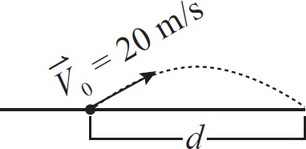5. A soccer ball on a level field is kicked at an angle of 30 degrees above the horizontal with an initial speed of 20 m/s, as drawn above. What is d, the distance from the spot of the kick to the spot where the ball lands? Ignore air resistance.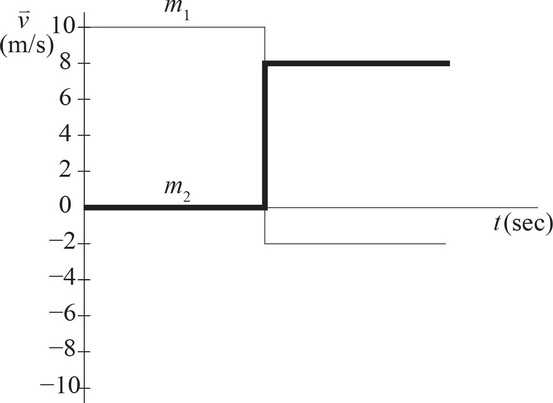6. Question below refers to the graph above.

Two objects of masses m1 and m2 undergo a collision. The graph above shows their velocities with respect to time both before and after the collision. If m1 = 10 kg, then m2 must be7. Question below refers to the graph above.

If the two objects have masses of m1 = 4 kg and m2 = 6 kg, what type of collision does the graph represent?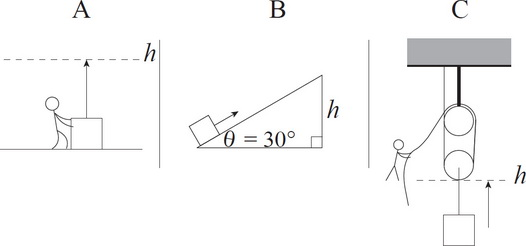8. The diagrams above show a box of mass m being lifted from the ground up to a height of h via three different methods. In situation A, the box is simply lifted by a person. In B, it is pushed up a ramp with an incline angle of 30 degrees. In C, it is lifted by a pulley system. Assuming ideal conditions (no friction) for all of these situations, which of the following correctly ranks the amount of work required to lift the box in each case?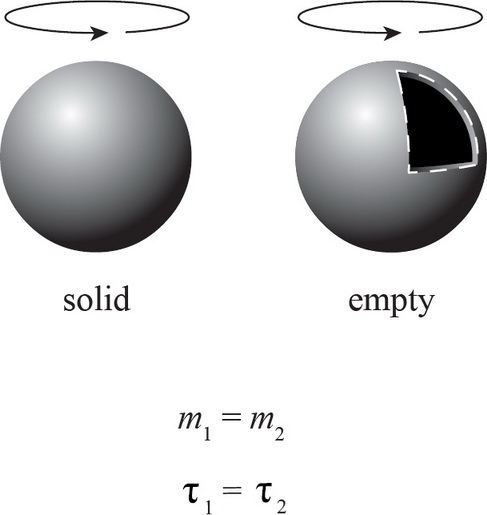9. Two spheres of equal size and equal mass are rotated with an equal amount of torque. One of the spheres is solid with its mass evenly distributed throughout its volume, and the other is hollow with all of its mass concentrated at the edges. If both spheres start at rest, which one is rotating faster after 1 second?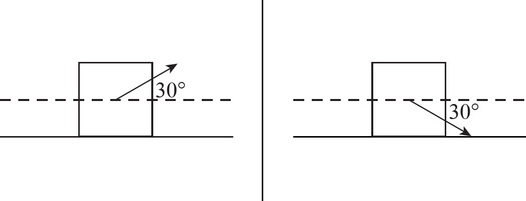10. Pulling a block of mass m to the right by a connected string at an angle of 30 degrees above the horizontal (as shown in the left picture) with a force equal to the block's weight produces a friction force Fkf. If the same block were to be pulled at an angle of 30 degrees beneath the horizontal (as shown in the right picture), what would be the friction force? Assume that the applied force is enough to make the block move in both cases.